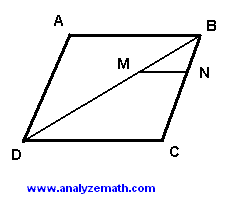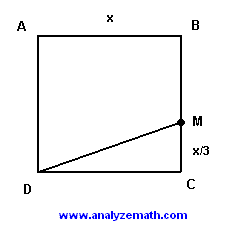# Free SAT Maths Questions with Solutions and Explanations - Sample 3

The solutions with explanations of the SAT maths questions in sample 3, are presented.

 If a2 - b2 = 30 and a - b = 5, then a = Solution Rewrite a2 - b2 = 30 as follows a2 - b2 = 30 (a - b)(a + b) = 30 Use the fact that a - b = 5 to find a + b a + b = 30 / (a - b) = 30 / 5 = 6 Add the right and left hand terms of the equations a - b = 5 and a + b = 6 to find (a - b) + (a + b) = 5 + 6 2a = 11 a = 11/2 points A(8,0) and B(0,6) are points in the standard coordinate plane. Let a be the area, in squared units, of a circle that passes through A and B. Which of the following is the set of all possible values of a? A) a < 25Pi B) a > 48 C) a > 10 D) a ≥ 100Pi E) a ≥ 25Pi Solution Since A and B are on the circle, the distance between them can be less than or equal to the diameter D = 2 R (R is the radius) of the circle. Hence 2 R ≥ sqrt( (82 - 0) + (0 - 62) ) Simplify R ≥ 5 Square both sides of the inequality and multiply them by Pi R2 ≥ 25 Pi R2 ≥ 25 Pi Since Pi R2 is the formula for the area a of the circle, then a ≥ 25 Pi If 4x + y = 5 and 2x + 3y = 12, then 3x + 2y = Solution Add the right hand sides and left hand sides of the two equations and simplify (4x + y) + (2x + 3y) = 5 + 12 6x + 4y = 17 Divide all terms of the above equation by 2 3x + 2y = 17/2 The price x of a t-shirt was first reduced by 10%. Then the new price was reduced by a further 20%. The price of the T-shirt after the two reductions is given by A) x - 0.3x B) x - 30 C) x - 0.3x + 0.02x D) x + 0.02x E) x + 0.3x Solution Price after first reduction x - 10% x = x - 0.1 x Reduction after the second reduction (x - 0.1x) - 20%(x - 0.1x) = x - 0.1x - 0.2x + 0.02x = x - 0.3x + 0.02x If x + y = √22 and (x - y)2 = 10, then xy = Solution Square both sides of the equation x + y = √22 (x + y)2 = (√22)2 Expand (x + y)2 and (x - y)2 in the above equations x2 + y2 + 2 xy = 22 x2 + y2 - 2 xy = 10 Subtract the left and right hand sides of the two equations above (x2 + y2 + 2 xy) - (x2 + y2 - 2 xy ) = 22 - 10 4 xy = 12 xy = 3 ABCD is a parallelogram and MN is parallel to to DC. The length of BN is 1/3 of the length of BC. What is the ratio of the area of triangle BNM to the area of the parallelogram ABCD?. Solution Since MN is parallel to DC, triangles BMN and BDC are similar and therefore BM / BD = BN / BC = 1/3 The area A1 of triangle MBN is given by A1 = (1/2)*sin(S)*BM*BN The area A2 of the parallelogram ABCD is twice the area of triangle DBC and is given by A2 = 2(1/2)*sin(S)*BD*BC = sin(S)*BD*BC The ratio A1 / A2 is given by A1 / A2 = (1/2)*sin(S)*BM*BN / sin(S)*BD*BC = (1/2)(BM/BD)(BN/BC) = (1/2)(1/3(1/3) = 1/18 If T = r R / (r + R), then R = Solution Cross product gives T(r + R) = r R Solve for R T r + T R = r R T R - r R = T r R(T - r) = - T r R = - T r / (T - r) R = T r / (r - T) If -2< x <2, then |4 - x2| / (x - 2) = Solution If -2< x <2 then -2 + 2 < x + 2 <2 + 2 0 < x + 2 < 4 and also -2 - 2 < x - 2 <2 - 2 -4 < x - 2 <0 |4 - x2| may be written as |4 - x2| = |2 - x||2 + x| = |x - 2||x + 2| Because of the inequalities 0 < x + 2 < 4 and -4 < x - 2 <0, we can write |x - 2||x + 2| = -(x - 2)(x + 2) Hence |4 - x2| / (x - 2) = -(x - 2)(x + 2)/(x - 2) = -(x + 2) For all real numbers x, |-x2 - 1| = Solution Rewrite as follows |-x2 - 1| = |-(x2 + 1)| Simplify = x2 + 1 If 3/7 of the students in a school are girls, what is the ratio of boys to girls in this school? Solution Let x be the total number of boys and girls in the school. The number of boys is given by x - (3/7) x = (4/7) x The ratio of boys to girls is given by [ (4/7) x ] / [ (3/7) x ] = 4/3 If 7/5 of a number is 6 more than 20% of the number, what is 11/10 of the number? Solution Let x be the number. The statement "7/5 of a number is 6 more than 20% of the number" is mathematically translated as follows (7/5) x = 20% x + 6 The above equation may be written as (7/5) x = (1/5) x + 6 Solve for x x = 5 (11/10) x = 55 / 10 = 5.5 What is the degree measure of the smaller angle formed by the hour and the minute hands of a clock at 2:50? Solution Each "5 minutes" division correspond to a central angle of size 360 / 12 = 30 degrees.. The angle corresponding to the fifth divison has a size equal to Minute hand: 60 minutes corresponds to 30 degrees (angle made by hour hand) 50 minutes corresponds to x x = 30 * 50 / 60 = 25 degrees The size of the angle is 4 * 30 + 25 = 145 degrees AC is a diameter of the circle shown below and B is a point on the circle such that triangle ABC is isosceles. If the circle has a circumference of 8 Pi, what is the area of the shaded region?. Solution The circumference is given, we can therefore find the radius R as follows 2 Pi R = 8 Pi R = 4 Since AC is the diameter of the circle, ABC is a right triangle (Thales theorem). We now use Pythagora's theorem to triangle ABC to find the length of AB and BC. AB2 + BC2 = 82 Triangle ABC is isosceles, hence AB = BC. Hence 2 AB2 = 64 ; AB2 = 32 AB = AC = 4 √ 2 Let As be the area of the shaded region. If we subtract the area of the right triangle ABC from the area of the semi circle, we obtain an area twice As. Hence 2 As = (1/2) Pi 42 - (1/2) AB AC = 8 Pi - 16 As = 4 Pi - 8 ABCD is a square of side x feet. MCD is a triangle such that MC = x/3 feet. Find x so that the area of the trapezoid ABMD is equal to 30 square feet.. Solution The area of the trapezoid is given by (1/2) x ( x + (2/3) x) = (x / 2)[ (3x + 2x) / 3 ] = (5/6) x2 The area of the trapezoid is known to be 30; hence (5 / 6) x2 = 30 x2 = 36 and x = 6 The average (arithmetic mean) of 3 numbers is 42. The average of another 5 numbers is 50. What is the average of all 8 numbers grouped together? A) 46 B) 47 C) 48 D) 49 E) 50 What is 5% of 6%? A) 0.3 B) 3 C) 0.03 D) 0.0003 E) 0.00003 At a high school, where each student takes one foreign language only, 3/8 of the students take French and 1/5 of the remaining students take German. If all the other students study Italian, what percent of all students take Italian? A) 50 B) 80 C) 10 D) 40 E) 30 On a certain day, a student spent 1/4 of his homework time reviewing his lessons, 7/12 of the time solving the assigned homework and the rest of the time revising for his coming tests. What is the ratio of the time spent revising for his tests to the time spent solving his assigned homework? A) 1:7 B) 1:3 C) 2:7 D) 7:12 E) 7:4 From 2003 to 2004 the profits made by a company increased by 10%. A year later in 2005, the profits of this company decreased to their 2003 value. By what percent did the profits decrease from 2004 to 2005? A) 10 B) 5 C) 1 D) 20 E) 9 In a bookshop, the sales of scientific books increased by 40% while the sales of engineering books decreased by 50% from 2001 to 2002. If R is the ratio of the number of scientific books to the number of engineering books in 2001 and r the same ratio in 2002, what is k if it is given by k = r/R? A) 2.8 B) 1.25 C) 0.2 D) 1 E) 0.8 If f(x - 2) = g(x + 1) + 1/x + 2, what does f(0) equal? A) g(1) + 2 B) undefined C) g(3) + 5/2 D) g(0) + 2 E) g(1) Which of the following is not equivalent to 7/10? A) 0.7 B) 35/50 C) (9/10)*(15/18) D) 70% E) 3/10 � 3/7 If 3x - 2 = 41, what is the value of √(3x + 6)? A) √(43/3) B) √49 C) √41 D) √43 E) √(41/43) If n and m are integers such that n2 = √(|m|) / 2, which of the follwowing CANNOT be the value of m? A) -4 B) 0 C) 64 D) -1024 E) 9 The system of equations 2x + y = 2 and -x + ky = 5 does not have a solution if k = A) 0 B) 1 C) 1/2 D) -1/2 E) 5 Answers to the Above Questions A E D C A B E E C B A D E C B D A C E A C C B E D More ACT and SAT practice